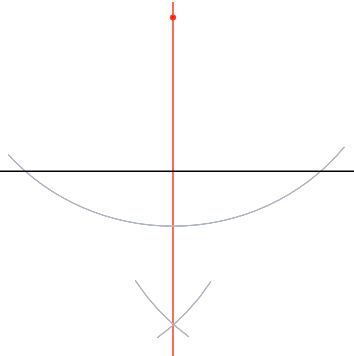Construction - Perpendicular from a point

## Construction - Perpendicular from a point

The perpendicular from a point to a line is the shortest distance from that point to that line.

To construct a perpendicular line from a given point above a base line, set the radius of the compass to be slightly more than the distance of the point to the base line.

Draw an arc with this setting to intersect the base line in two places.

Without changing the radius of the compass, set the point on one of the base line intersections and draw an arc below the base line. Repeat for the other intersection.

Draw a perpendicular line from that intersection through the original point.

## Example 1

Draw an arc from a point above a horizontal line.

How to do it: from the point, draw an arc to cut through the line in two places.Without changing the compasses, draw new arcs below the line from each intersection point.The line connecting the point and the intersection of the arcs is a perpendicular line.